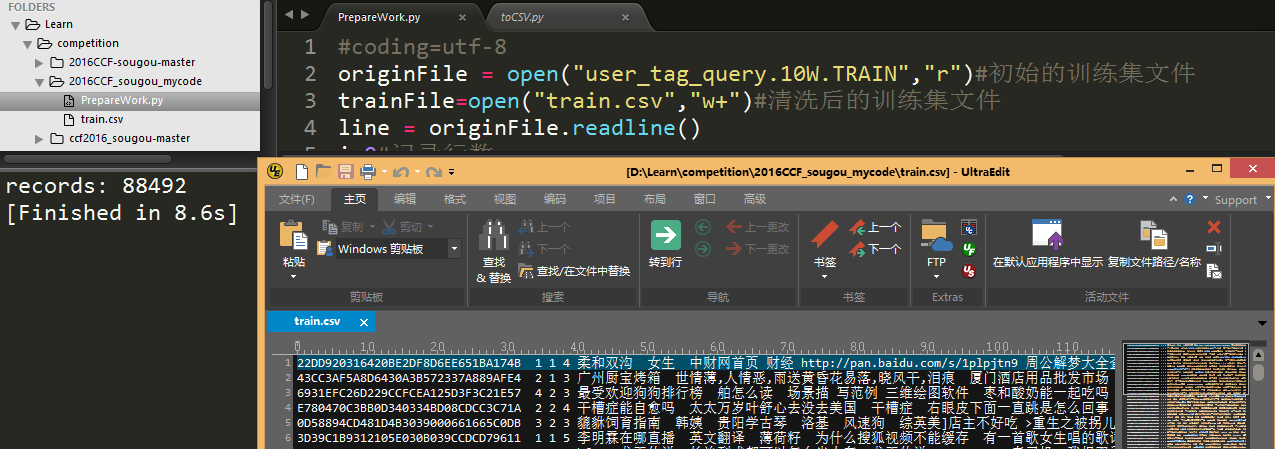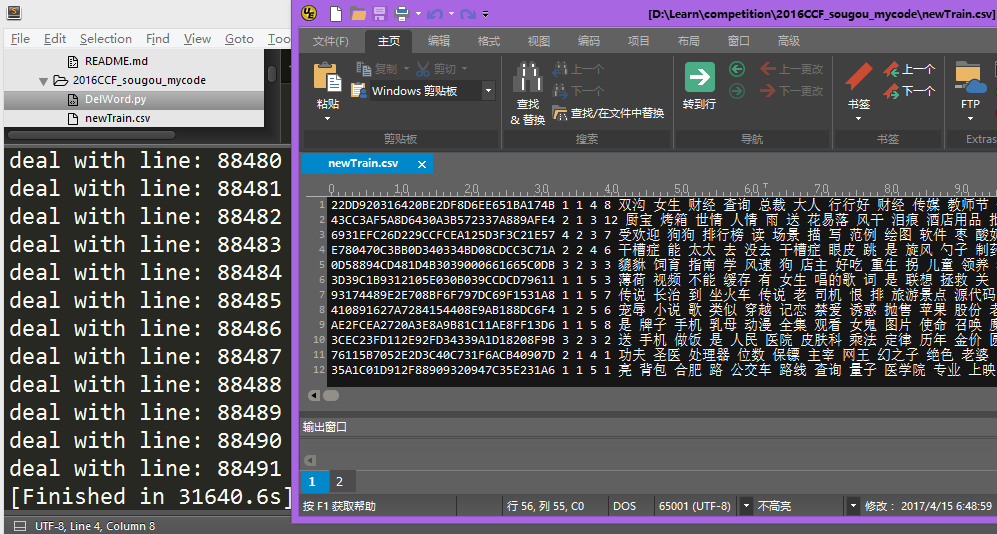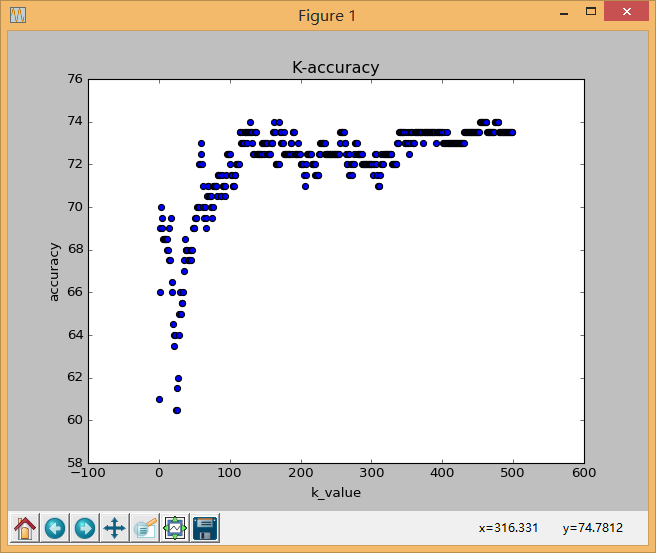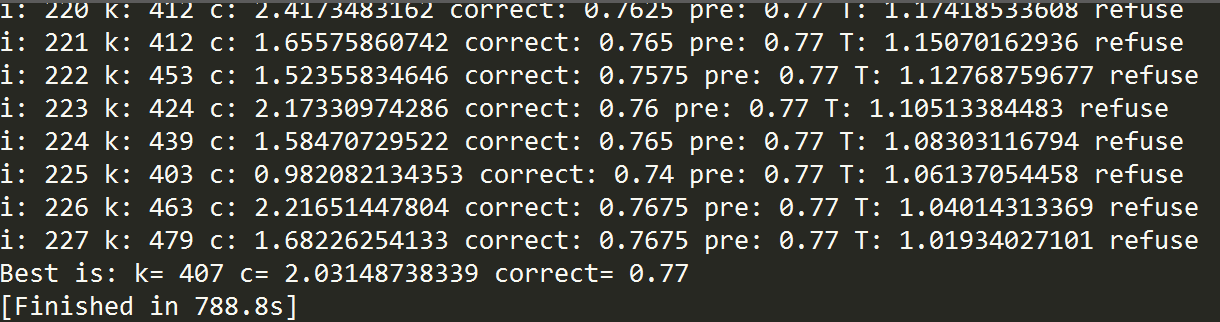# 使用sklearn进行文本分类(以搜狗用户画像为例)

### 2.数据预处理

#### (1)数据清洗

user_tag_query.10W.TRAIN编码方式为GB18030，先将其转换为UTF-8编码，可使用文本编辑器转码，这里使用python对文件转码。

#coding=utf-8
originFile = open("user_tag_query.10W.TRAIN","r")#初始的训练集文件
trainFile=open("train.csv","w+")#清洗后的训练集文件
i=0#记录行数
count=0#记录有效数据数
while line:
i+=1
datas = line.split("\t")

if datas!='0' and datas!='0' and datas!='0':#只记录没有标签缺失的用户
count+=1
context='\t'.join(datas[0:4])+'\t'+'\t'.join(datas[4:]).decode('GB18030').encode('UTF-8')
trainFile.write(context)

print "records:",count
originFile.close()#### (2)分词及筛选

#encoding=utf-8
import jieba
import jieba.posseg
import sys

sys.setdefaultencoding('utf8')

originFile = open("train.csv","r")#初始的训练集文件
trainFile=open("newTrain.csv","w")#处理后的训练集文件

usefulWord=['n','v']#保存词性
q=0#行数

while line:
if q%1==0:
print 'deal with line:',q
q+=1

inputStr = line.split("\t")
count=0#记录空格
userSaveWord=[]#记录用户的有效单词
context=' '.join(inputStr[0:4])#保存用户标签信息

for i in inputStr[4:]:
words=jieba.posseg.cut(i)
for w in words:
if w.word==' ':
count+=1
continue
for u in usefulWord:
if w.flag==u:
userSaveWord.append(w.word.encode('UTF-8'))
break
else:
continue

context+=' '+str(count)+' '+' '.join(userSaveWord[:])+'\n'
trainFile.write(context)### 3.特征选择

#coding=utf-8
from numpy import *

originFile = open("newTrain.csv","r")#初始的训练集文件
trainFile=open("info.csv","w")#清洗后的训练集文件

age=[0,0,0,0,0,0]
sex=[0,0]
edu=[0,0,0,0,0,0]

while line:
datas = line.split(" ")
a=datas;s=datas;e=datas
#print a,s,e
age[int(a)-1]+=1;sex[int(s)-1]+=1;edu[int(e)-1]+=1

trainFile.write(str(age)+"\n")
trainFile.write(str(sex)+"\n")
trainFile.write(str(edu)+"\n")

print "age:",age
print "sex:",sex
print "edu:",edu

```age: [35965, 24785, 16561, 8967, 2039, 175]
sex: [51540, 36952]
edu: [354, 556, 18708, 27956, 36852, 4066]
[Finished in 4.4s]```

# coding:utf-8
import sys
from numpy import *
import os
from sklearn import feature_extraction
from sklearn.feature_extraction.text import TfidfTransformer
from sklearn.feature_extraction.text import CountVectorizer
from sklearn.feature_selection import SelectKBest
from sklearn.feature_selection import chi2
from sklearn import svm
import matplotlib.pyplot as plt   #导入pyplot子库

sys.setdefaultencoding('utf8')

originFile = open("newtrain.csv","r")#初始的训练集文件

q=0
corpus=[]
target=[]

while line and q<l:
tmpDatas=line.split(' ')
datas=' '.join(tmpDatas[5:])
corpus.append(datas)
target.append(tmpDatas)

q+=1

vectorizer=CountVectorizer()#该类会将文本中的词语转换为词频矩阵，矩阵元素a[i][j] 表示j词在i类文本下的词频
wordCount=vectorizer.fit_transform(corpus)#将文本转为词频矩阵
wordList=vectorizer.get_feature_names()#获取词袋模型中的所有词语

originFile.close()
return corpus,target,wordCount,wordList

def chi(wordCount,target,k=200):#返回被选的k个特征构成的用户矩阵

res=SelectKBest(chi2, k).fit_transform(wordCount.toarray(), target)
return res

def test(l=1000,k=200,j=200,wordCount=None,target=None):#得到预测正确数与错误数

res=chi(wordCount,target,j)
X=res[:-k]
y=target[:-k]

clf = svm.SVC()
clf.fit(X, y)
result = clf.predict(res[-k:])

yes=0
no=0
for i in range(len(result)):
if target[i+l-k] == result[i]:
yes+=1
else:
no+=1

return yes,no

yes,no=0,0
l,k,j=1000,200,1

ax=[]
ay=[]

for i in range(500):
yes,no=test(l,k,j+i,wordCount,target)
score=yes*100.0/(yes+no)
print str(j+i)+"\t"+str(yes)+"\t"+str(no)+"\t"+str(score)
ax.append(i)
ay.append(score)

fig=plt.figure()
pp.scatter(ax,ay,s=30)
plt.ylabel('accuracy')  #给y轴添加标签
plt.xlabel('k_value')  #给x轴加标签
plt.title("K-accuracy");  #给2D图加标题
plt.show()  #显示2D图1.有多个属性的年龄、学历如何分类？
2.如何对10w用户做文本分类？
3.如何提高分类的准确性？
4.如何整理已完成的代码？

### 4.分类

#encoding=utf-8
import PrepareWork as p0
import DelWord as p1
import CountInfo as p2
import Classify as p3
#p0.InitOriginFile("user_tag_query.10W.TRAIN","train.csv")
#p1.DeleteStopWord("train.csv","newTrain.csv")
#p2.CountUserRate("newTrain.csv","info.csv")

### 5.优化

1.切分所有用户的所有语句成为单词，并将这些单词加入词袋
2.使用卡方检验选出K个最具有表现力的单词表示每一个用户
3.将用户中K个单词的出现次数组成向量，输入到SVM分类器
4.使用SVM预测测试集的性别

K值我们已经了解了，就是指选择的特征维数，那么C是什么。
C是SVM的惩罚参数，规定核函数与训练数据的拟合程度，参考sklearn关于svm参数说明文档

K是整数，或许还能使用穷举法求出，但是C是浮点数，又如何选择好的C参数提高分类的准确程度呢？
C的取值问题其实类似于函数求极值。试想我们假设K是固定的，只是为了寻找一个C使得SVM预测结果correct提升，把svm看做一个函数，C的取值就转换为求SVM函数的最大值问题。求函数极值方法有很多，这里我们使用模拟退火算法（可参考这篇文章大白话解析模拟退火算法

1.确定最大步长step(x每次前进或后退的最大值)，x取值范围L(x在区间L内取值)，取历史最高值时X的取值BestX，y的取值BestScore取最高温度T，取最低温度minT
2.随机选一个x初始值，
3.x按照步长step前进(x+step)或后退(x-step)得到x1
4.比较前进或后退时的y值是否增大，

5.T减小，如果T大于minT则重复3操作，否则停止
6.输出BestX和BestScore

def Annealing(self,minTemp=0,minScore=100,KSTEP=5,CSTEP=2):
#搜索的最大范围
KMAX,CMAX=2000,1000

#冷却表参数
MarkovLength = 10000;         #马可夫链长度
DecayScale = 0.98             #衰减参数
Temperature = 100             #初始温度
PreK,NextK=0,0                #prior and next value of x
PreC,NextC=0.0,0.0            #prior and next value of y
BestK,BestC=0,0.0             #最终解
PreScore,BestScore,Score=0.0,0.0,0.0#历史成绩，最好成绩，当前成绩
maxValue=1000000

#随机选点
PreK=1#K值
PreC=0#C值
PreBestK = BestK = PreK
PreBestC = BestC = PreC

yes,no=0,0
i=0
#温度过低或分数达到要求时停止
while Temperature>minTemp and BestScore<minScore:

Kstep,Cstep=maxValue,maxValue
NextK,NextC=PreK+Kstep,PreC+Cstep

#如果下一步越界了，重新定步长
while NextK>KMAX or NextK<=0 or NextC>CMAX or NextC<0:
Kstep=r.randint(0,KSTEP)-KSTEP/2
Cstep=CSTEP*(r.random()-0.5)
NextK,NextC=PreK+Kstep,PreC+Cstep

yes,no,Score=self.CalScore(NextK,NextC)
print "i:",i,"k:",NextK,"c:",NextC,"correct:",Score,"pre:",PreScore,"T:",Temperature,
if Score > PreScore :#比上一个解更好，接受
if Score>BestScore:#比最好解还好，替换
BestScore=Score
BestK=NextK
BestC=NextC
PreK=NextK
PreC=NextC
PreScore=Score
print "accept1"
else:#比上一个解差
change=1000.0*(Score-PreScore)/Temperature#降低的数值(转化为正数)
#print change,m.exp(change),
if m.exp(change) >r.random():#概率接受较差解
PreK=NextK
PreC=NextC
PreScore=Score
print "accept2"
else:#不接受
print "refuse"
pass
Temperature*=DecayScale
i+=1

return BestK,BestC,BestScore

#encoding=utf-8
import PrepareWork as p0
import DelWord as p1
import CountInfo as p2
import Classify as p3
#p0.InitOriginFile("user_tag_query.10W.TRAIN","train.csv")
#p1.DeleteStopWord("train.csv","newTrain.csv")
#p2.CountUserRate("newTrain.csv","info.csv")

def testAnn():
k,c,score=clf.Annealing(1,80,100,2)
print "Best is:","k=",k,"c=",c,"correct=",score

def testClassify():
global yes,no
for i in range(1,2000):
yes,no=clf.test(i*10)
print "k:",i*10,"yes:",yes,"no:",no,"correct:",yes*1.0/(yes+no)

yes,no=0,0
allNum,testNum=2000,400
k,c,score=1,1.0,0
allNum,testNum=2000,400
clf=p3.Clf(allNum,testNum)

testAnn()Thanks~

## 发布者